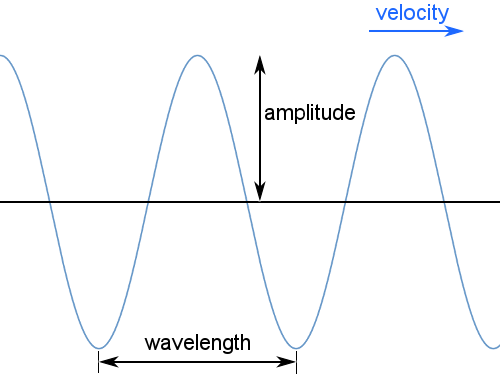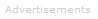# Definition of Wave

What is a Wave?

A wave is a physical phenomenon characterized by its frequency, wavelength, and amplitude.

In general, waves transfer energy from one location to another, in which case they have a velocity.Standing waves may also occur; these have no net velocity and involve no net transfer of energy. They have frequency, wavelength and amplitude.

Mechanical waves require a medium to travel through: for example, sound waves and earthquake waves cannot travel through a vacuum.

Electromagnetic waves, such as light, do not require a medium and can travel through a vacuum.

Transverse waves, such as light, oscillate perpendicular to the direction the wave is carrying energy in - as in the diagram above. Longitudinal waves, such as sound, oscillate parallel to the direction of energy transfer.

Properties

Classical wave properties include reflection, refraction, and diffraction.Wave-Particle Duality

Quantum theory established the principle of wave-particle duality or the matter wave: particles may behave like waves with velocities, wavelengths, amplitudes, and frequencies; and can be reflected, refracted and diffracted.

Electron Diffraction

Electron diffraction methods are important in determining the structures of solids.

The de Broglie Equation

The wavelength of matter waves can be calculated from the de Broglie equation:

λ = h / p

where: λ = wavelength; h = Planck's constant; and p = momentum.

The higher the mass of a particle, the shorter its wavelength. In practice, only atomic-scale masses and smaller have observable wavelengths.

The Dirac Equation

The Dirac equation, a wave equation for electrons travelling at speeds close to the speed of light, naturally produces the quantum mechanical property of electron spin.Search the Dictionary for More Terms